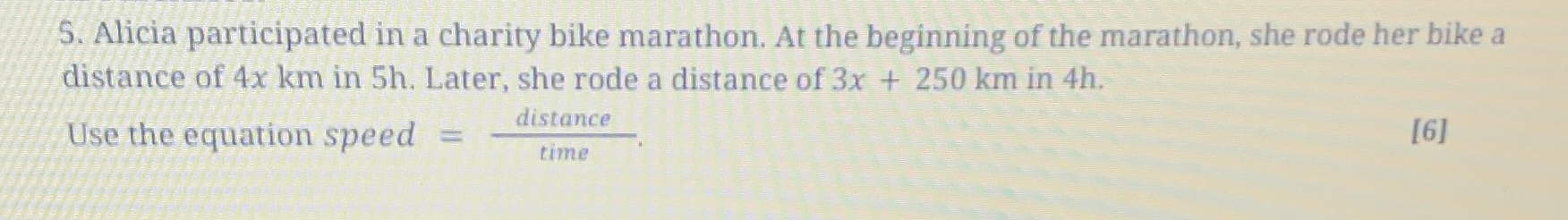### ¿Todavía tienes preguntas de matemáticas?

Pregunte a nuestros tutores expertos
Algebra
Pregunta5. Alicia participated in a charity bike marathon. At the beginning of the marathon, she rode her bike a distance of $$4 x km$$ in $$5 h$$ . Later, she rode a distance of $$3 x + 250 km$$ in $$4 h$$ . Use the equation speed $$= \frac{distance}{time}$$.